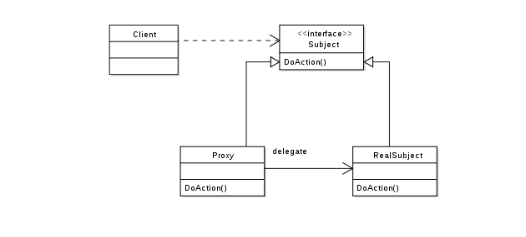# 面试官提问：什么是动态代理？

fatherlaw

0收藏•  接口类

``````public interface Calculator {

/**
* 计算两个数之和
* @param num1
* @param num2
* @return
*/
}``````

•  目标对象

``````public class CalculatorImpl implements Calculator {

@Override
public Integer add(Integer num1, Integer num2) {
Integer result = num1 + num2;
return result;
}
}``````

•  代理对象

``````public class CalculatorProxyImpl implements Calculator {

private Calculator calculator;

@Override
public Integer add(Integer num1, Integer num2) {
//方法调用前，可以添加其他功能....
//方法调用后，可以添加其他功能....
return result;
}

public CalculatorProxyImpl(Calculator calculator) {
this.calculator = calculator;
}
}``````

•  测试类

``````public class CalculatorProxyClient {

public static void main(String[] args) {
//目标对象
Calculator target = new CalculatorImpl();
//代理对象
Calculator proxy = new CalculatorProxyImpl(target);
System.out.println("相加结果：" + result);
}
}``````

•  输出结果

``相加结果：3``

3.1、JDK 中生成代理对象的玩法
•  创建接口

``````public interface JdkCalculator {

/**
* 计算两个数之和
* @param num1
* @param num2
* @return
*/
}``````

•  目标对象

``````public class JdkCalculatorImpl implements JdkCalculator {

@Override
public Integer add(Integer num1, Integer num2) {
Integer result = num1 + num2;
return result;
}
}``````

•  动态代理对象

``````public class JdkProxyFactory {

/**
* 维护一个目标对象
*/
private Object target;

public JdkProxyFactory(Object target) {
this.target = target;
}

public Object getProxyInstance(){
target.getClass().getInterfaces(),
new InvocationHandler(){

@Override
public Object invoke(Object proxy, Method method, Object[] args) throws Throwable {
System.out.println("方法调用前，可以添加其他功能....");

// 执行目标对象方法
Object returnValue = method.invoke(target, args);
System.out.println("方法调用后，可以添加其他功能....");
return returnValue;
}
});
return proxyClassObj;
}
}``````

•  测试类

``````public class TestJdkProxy {

public static void main(String[] args) {
//目标对象
JdkCalculator target = new JdkCalculatorImpl();
System.out.println(target.getClass());
//代理对象
JdkCalculator proxyClassObj = (JdkCalculator) new JdkProxyFactory(target).getProxyInstance();
System.out.println(proxyClassObj.getClass());
//执行代理方法
System.out.println("相加结果：" + result);
}
}``````

•  输出结果

``````class com.example.java.proxy.jdk1.JdkCalculatorImpl
class com.sun.proxy.\$Proxy0

``````1. 首先定义一个 InvocationHandler 实例，它负责实现接口的方法调用
2. 通过 Proxy.newProxyInstance() 创建 interface 实例，它需要 3 个参数:
（2）需要实现的接口数组，至少需要传入一个接口进去；
（3）用来处理接口方法调用的 InvocationHandler 实例。
3. 将返回的 Object 强制转型为接口``````

``````public class JdkCalculatorDynamicProxy implements JdkCalculator {

private InvocationHandler handler;

public JdkCalculatorDynamicProxy(InvocationHandler handler) {
this.handler = handler;
}

public void add(Integer num1, Integer num2) {
handler.invoke(
this,
new Object[] { num1, num2 });
}
}``````

3.2、cglib 生成代理对象的玩法

cglib 特点如下：

•  cglib 不仅可以代理接口还可以代理类，而 JDK 的动态代理只能代理接口
•  cglib 是一个强大的高性能的代码生成包，它广泛的被许多 AOP 的框架使用，例如我们所熟知的 Spring AOP，cglib 为他们提供方法的 interception（拦截）。
•  CGLIB包的底层是通过使用一个小而快的字节码处理框架ASM，来转换字节码并生成新的类，速度非常快。

``````<dependency>
<groupId>cglib</groupId>
<artifactId>cglib</artifactId>
<version>3.2.5</version>
</dependency>``````

•  创建接口

``````public interface CglibCalculator {

/**
* 计算两个数之和
* @param num1
* @param num2
* @return
*/
}``````

•  目标对象

``````public class CglibCalculatorImpl implements CglibCalculator {

@Override
public Integer add(Integer num1, Integer num2) {
Integer result = num1 + num2;
return result;
}
}``````

•  动态代理对象

``````public class CglibProxyFactory implements MethodInterceptor {

/**
* 维护一个目标对象
*/
private Object target;

public CglibProxyFactory(Object target) {
this.target = target;
}

/**
* 为目标对象生成代理对象
* @return
*/
public Object getProxyInstance() {
//工具类
Enhancer en = new Enhancer();
//设置父类
en.setSuperclass(target.getClass());
//设置回调函数
en.setCallback(this);
//创建子类对象代理
return en.create();
}

@Override
public Object intercept(Object o, Method method, Object[] args, MethodProxy methodProxy) throws Throwable {
System.out.println("方法调用前，可以添加其他功能....");

// 执行目标对象方法
Object returnValue = method.invoke(target, args);
System.out.println("方法调用后，可以添加其他功能....");
return returnValue;
}
}``````

•  测试类

``````public class TestCglibProxy {

public static void main(String[] args) {
//目标对象
CglibCalculator target = new CglibCalculatorImpl();
System.out.println(target.getClass());
//代理对象
CglibCalculator proxyClassObj = (CglibCalculator) new CglibProxyFactory(target).getProxyInstance();
System.out.println(proxyClassObj.getClass());
//执行代理方法
System.out.println("相加结果：" + result);
}
}``````

•  输出结果

``````class com.example.java.proxy.cglib1.CglibCalculatorImpl

``````public class CglibCalculatorImplByCGLIB extends CglibCalculatorImpl implements Factory {

private static final MethodInterceptor methodInterceptor;

private static final Method method;

public final Integer add(Integer var1, Integer var2) {
return methodInterceptor.intercept(this, method, new Object[]{var1, var2}, methodProxy);
}

//....
}``````

•  创建新的目标对象

``````public class CglibCalculatorClass {

/**
* 计算两个数之和
* @param num1
* @param num2
* @return
*/
public Integer add(Integer num1, Integer num2) {
Integer result = num1 + num2;
return result;
}
}``````

•  测试类

``````public class TestCglibProxyClass {

public static void main(String[] args) {
//目标对象
CglibCalculatorClass target = new CglibCalculatorClass();
System.out.println(target.getClass());
//代理对象
CglibCalculatorClass proxyClassObj = (CglibCalculatorClass) new CglibProxyFactory(target).getProxyInstance();
System.out.println(proxyClassObj.getClass());
//执行代理方法
System.out.println("相加结果：" + result);
}
}``````

•  输出结果

``````class com.example.java.proxy.cglib1.CglibCalculatorClass
class com.example.java.proxy.cglib1.CglibCalculatorClass\$\$EnhancerByCGLIB\$\$e68ff36c

•  方法调用前拦截
•  方法调用后拦截
•  调用方法结束拦截
•  抛出异常拦截

``````<plugin>
<groupId>org.codehaus.mojo</groupId>
<artifactId>aspectj-maven-plugin</artifactId>
<version>1.5</version>
<executions>
<execution>
<goals>
<goal>compile</goal>
<goal>test-compile</goal>
</goals>
</execution>
</executions>
<configuration>
<source>1.6</source>
<target>1.6</target>
<encoding>UTF-8</encoding>
<complianceLevel>1.6</complianceLevel>
<verbose>true</verbose>
<showWeaveInfo>true</showWeaveInfo>
</configuration>
</plugin>``````

``````@RequestMapping({"/hello"})
public String hello(String name) {
String result = "Hello World";
System.out.println(result);
return result;
}``````

``````@Aspect
public class ControllerAspect {

/***
* 定义切入点
*/
@Pointcut("execution(* com.example.demo.web..*.*(..))")
public void methodAspect(){}

/**
* 方法调用前拦截
*/
@Before("methodAspect()")
public void before(){
System.out.println("代理 -> 调用方法执行之前......");
}

/**
* 方法调用后拦截
*/
@After("methodAspect()")
public void after(){
System.out.println("代理 -> 调用方法执行之后......");
}

/**
* 调用方法结束拦截
*/
@AfterReturning("methodAspect()")
public void afterReturning(){
System.out.println("代理 -> 调用方法结束之后......");
}

/**
* 抛出异常拦截
*/
@AfterThrowing("methodAspect()")
public void afterThrowing() {
System.out.println("代理 -> 调用方法异常......");
}
}``````

``````@RequestMapping({"/hello"})
public String hello(Integer name) throws SQLException {
JoinPoint var2 = Factory.makeJP(ajc\$tjp_0, this, this, name);

Object var7;
try {
Object var5;
try {
//调用before
String result = "Hello World";
System.out.println(result);
var5 = result;
} catch (Throwable var8) {
Aspectj.aspectOf().after(var2);
throw var8;
}
//调用after
Aspectj.aspectOf().after(var2);
var7 = var5;
} catch (Throwable var9) {
//调用抛出异常
Aspectj.aspectOf().afterthrowing(var2);
throw var9;
}
//调用return
Aspectj.aspectOf().afterRutuen(var2);
return (String)var7;
}``````

•  如果目标是接口的话，默认使用 JDK 的动态代理技术；
•  如果目标是类的话，使用 cglib 的动态代理技术；
•  引入了AspectJ中的一些注解@pointCut、@after，@before，主要是为了简化使用，跟AspectJ的关系并不大；

1、Java三种代理模式：静态代理、动态代理和cglib代理

2、Java 动态代理作用是什么？